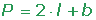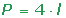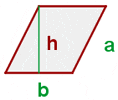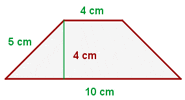Learn from home

The teachers## Perimeter

The perimeter of a polygon is equal to the sum of the length of its sides.

## Area

The area of a polygon is the measure of the region enclosed by the sides of a polygon.

## Perimeter of a Triangle

 Equilateral Triangle Isosceles Triangle Scalene Triangle### Area of a Triangle## Example

Find the area and perimeter of the following triangle:P = 2 · 11 + 7.5 = 29.5 cm### Square## Example

Calculate the area and perimeter of a square with 5 cm sides.A = 5² = 25 cm²

### Rectangle## Example

Calculate the area and perimeter of a rectangular with a base of 10 cm and a height of 6 cm.P = 2 · (10 + 6) = 32 cm

A = 10 · 6 = 60 cm²

### Rhombus## Example

Calculate the area and perimeter of a rhombus whose diagonals are 30 and 16 cm, and its side measures 17 cm.P = 4 · 17 = 68 cm### RhomboidP = 2 · (a + b)

A = b · h

## Example

Calculate the area and perimeter of a rhomboid shape of 4 sides of 4.5 cm and a height of 4 cm.P = 2 · (4.5 + 4) = 17 cm

A = 4 · 4 = 16 cm²

### Area of a Trapezoid## Example

Calculate the area of the following trapezoid:### Area of a Regular Polygonn is the number of sidesCalculate the area and perimeter of a regular pentagon with sides of 6 cm.By applying the Pythagorean theorem for one of the triangles, we obtain:Calculate the area and perimeter of a regular hexagon inscribed in a circle of 4 cm radius.P = 6 · 4 = 24 cm### Area of a PolygonThe area is obtained by triangulating the polygon and adding the area of these triangles.

A = T1 + T2 + T3 + T4

Calculate the area of the following polygon:P = 11 · 2 + 5 + 13 + 12 = 52 cm

AD = BC; AB = DCRhomboid

A = AR + AT

A = 11 · 12 + (12 · 5 ) : 2 = 162 cm²

Did you like the article?(4 votes, average: 4.00 out of 5)Loading...

Emma

I am passionate about travelling and currently live and work in Paris. I like to spend my time reading, gardening, running, learning languages and exploring new places.

Did you like
this resource?

Bravo!